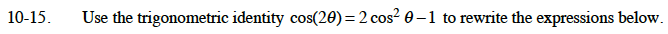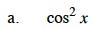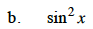### Home > CALC > Chapter 10 > Lesson 10.1.2 > Problem10-15

10-15.$2\cos^2(x)=\cos(2x)+1$$\cos^2(x)=1-\sin^2(x)$

$\cos(2x)=2(1-\sin^2(x))-1$

Now solve for sin2(x).